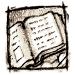MA010 Graph Theory (an online guide)

# Lecture 9*: Intersection graphs in brief

Outline

•Intersection graphs (of objects or sets)
definition of an intersection graph of a set family, interval graphs as an example
• Chordal graphs and their properties
definition, simplicial decomposition, chordal graphs as intersection graphs of subtrees in a tree
• Some special classes of intersection graphs
line graphs, interval graphs, circle graphs, disc graphs, etc, contact graphs,
string and segment graphs

Goals

This lecture introduces some basic concepts of intersection representations of graphs, and of so called "graph classes" topics. Intersection graphs are mostly related to geometric objects, but some abstract results are also provided. For instance, the chordal graphs turn out to be very important in other parts of graphs theory (see Lecture 10*). Some parts of this lecture are also related to planarity (Lecture 8).Remark (repeated)
Subsequent lectures (nine to higher) present, time depending, some additional interesting graph material which will not be examined directly (though its knowledge will also be helpful at the final exam). The contents of these additional lectures may vary from year to year, and not all extra topics outlined in this syllabus will be presented.

Generally, the additional topics are not covered at all by the course textbooks, and only online materials are presented.